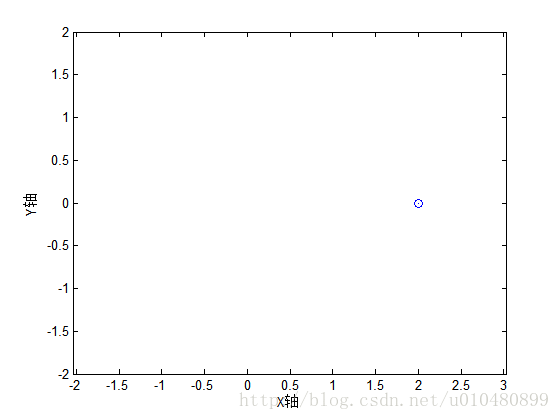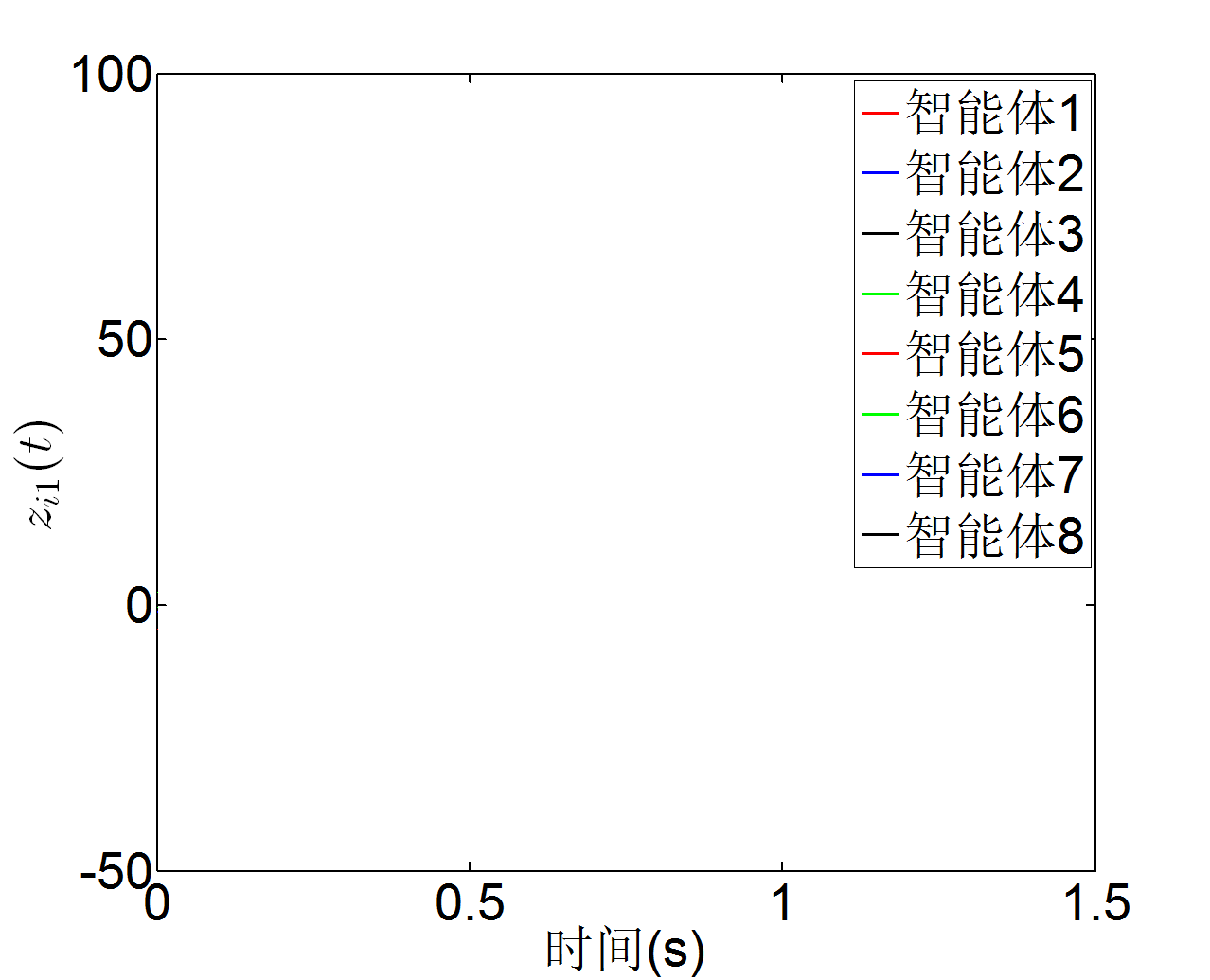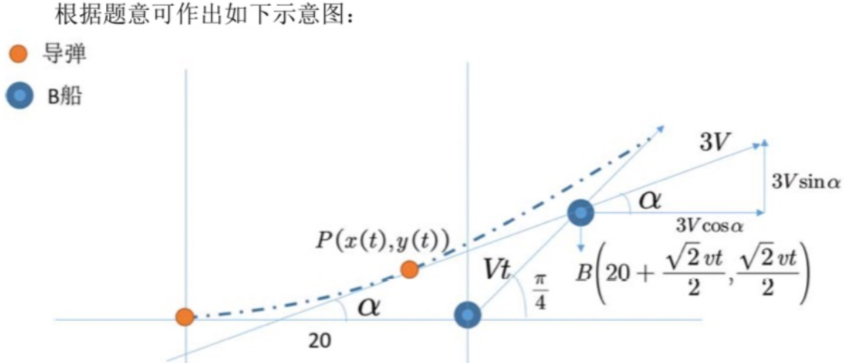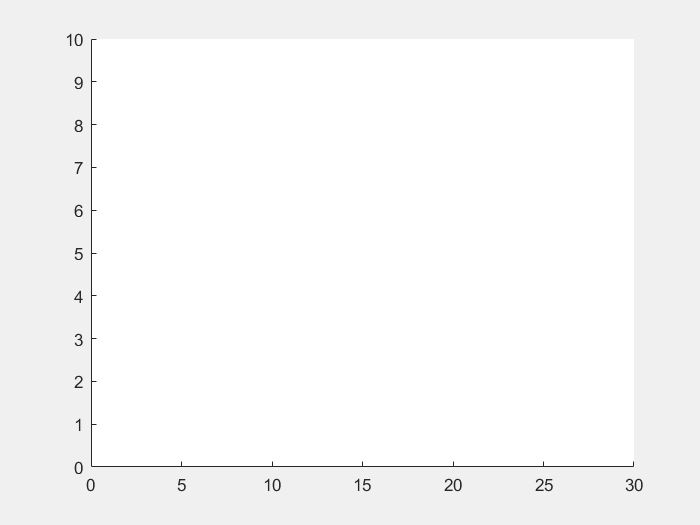• 2021-04-19 07:47:47

第一种方式

close all;

clear all;

clc;

clf;

xlabel('X轴');

ylabel('Y轴');

box on;

axis([-2,2,-2,2]);

axis equal;

pause(1);

h=line(NaN,NaN,'marker','o','linesty','-','erasemode','none');

t=6*pi*(0:0.02:1);

for n=1:length(t)

set(h,'xdata',2*cos(t(1:n)),'ydata',sin(t(1:n)));

pause(0.05);

frame=getframe(gcf);

imind=frame2im(frame);

[imind,cm] = rgb2ind(imind,256);

if n==1

imwrite(imind,cm,'test.gif','gif', 'Loopcount',inf,'DelayTime',1e-4);

else

imwrite(imind,cm,'test.gif','gif','WriteMode','append','DelayTime',1e-4);

end

end

效果图close all;

clear all;

clc;

clf;

%白色背景

axis([-2,2,-2,2]);

xlabel('X轴');

ylabel('Y轴');

%四周的边框

box on;

%绘图区域

t=0:0.02:10;

Nt=size(t,2);

x=2*cos(t(1:Nt));

y=sin(t(1:Nt));

%循环绘图

for i=1:Nt;

cla;

hold on;

plot(x,y)

plot(x(i),y(i),'o');

frame=getframe(gcf);

imind=frame2im(frame);

[imind,cm] = rgb2ind(imind,256);

if i==1

imwrite(imind,cm,'test.gif','gif', 'Loopcount',inf,'DelayTime',1e-4);

else

imwrite(imind,cm,'test.gif','gif','WriteMode','append','DelayTime',1e-4);

end

end

效果图

更多相关内容
• ## Matlab绘制动态图

千次阅读 2021-05-20 10:25:07
在写论文的过程中，我们经常需要用MATLAB绘制图形。论文中的图形都是图片格式，但是在展示和汇报时，如果将图形做成GIF动图，变量的变化过程就会非常直观，展示效果也会非常好。下面将本人利用MATLAB绘制GIF动图的...

在写论文的过程中，我们经常需要用MATLAB绘制图形。论文中的图形都是图片格式，但是在展示和汇报时，如果将图形做成GIF动图，变量的变化过程就会非常直观，展示效果也会非常好。下面将本人利用MATLAB绘制GIF动图的方法分享给大家。

• 前提：已经利用MATLAB完成了图片的绘制，保存有变量数据

具体程序如下所示：

figure(1)
clf;
box on;
%坐标轴信息
xlabel({'时间(s)'},'FontSize',40)
ylabel({'$z_{i1}(t)$'},'Interpreter','latex','FontSize',40)
%设置坐标轴线宽
set(gca,'LineWidth',2,'FontSize',40)
%坐标显示范围
axis([0,1.5,-50,100])
legend('智能体1','智能体2','智能体3','智能体4','智能体5','智能体6','智能体7','智能体8');
pausetime=0.001; %设置绘图速度
pause(1);
%绘制八条曲线，设置每条曲线的颜色和线宽
h1=line(NaN,NaN,'color','r','linewidth',2.5);
h2=line(NaN,NaN,'color','b','linewidth',2.5);
h3=line(NaN,NaN,'color','k','linewidth',2.5);
h4=line(NaN,NaN,'color','g','linewidth',2.5);
h5=line(NaN,NaN,'color','r','linewidth',2.5);
h6=line(NaN,NaN,'color','g','linewidth',2.5);
h7=line(NaN,NaN,'color','b','linewidth',2.5);
h8=line(NaN,NaN,'color','k','linewidth',2.5);
t=1*(0.001:0.001:1.5);
%直接用n=1:length(t)，绘制速度超级慢；n=1:20:length(t) ,中间的20表示多久取一次样，可以调节绘制速度
for n=1:20:length(t)
%z1,z2,...,z8是之前MATLAB绘图时，保存的变量，也就是前提条件中的绘制图形保存的变量
set(h1,'xdata',t(1:n),'ydata',z1(1,1:n));%设置质点1的运动
set(h2,'xdata',t(1:n),'ydata',z2(1,1:n));%设置质点2的运动
set(h3,'xdata',t(1:n),'ydata',z3(1,1:n));%设置质点3的运动
set(h4,'xdata',t(1:n),'ydata',z4(1,1:n));%设置质点4的运动
set(h5,'xdata',t(1:n),'ydata',z5(1,1:n));%设置质点5的运动
set(h6,'xdata',t(1:n),'ydata',z6(1,1:n));%设置质点6的运动
set(h7,'xdata',t(1:n),'ydata',z7(1,1:n));%设置质点7的运动
set(h8,'xdata',t(1:n),'ydata',z8(1,1:n));%设置质点8的运动
pause(pausetime);
legend('智能体1','智能体2','智能体3','智能体4','智能体5','智能体6','智能体7','智能体8');
drawnow;
%下面是保存为GIF的程序
frame=getframe(gcf);
imind=frame2im(frame);
[imind,cm] = rgb2ind(imind,256);
if n==1
imwrite(imind,cm,'Z1.gif','gif', 'Loopcount',inf,'DelayTime',1e-4);
else
imwrite(imind,cm,'Z1.gif','gif','WriteMode','append','DelayTime',0);
end
end


GIF绘制效果如下所示：展开全文• matalab时频绘制，可以轻松的帮助你绘制时频，有兴趣的可以下载
• ## matlab绘制动态图

千次阅读 2020-06-29 10:11:59
常用的包括两种方式：plot方式及drawnow方式 ...一定循环次数后一下移动后的点（若想看动态效果一定要pause一小段时间） 代码 clc;clear DeltaT = 0.0000001; n = 1000000000; % 导弹最长运行时间10

常用的包括两种方式：plot方式及drawnow方式
以导弹跟踪问题为例子。
位于坐标原点的A船向位于其正东⽅20个单位的B 船发射导弹，导弹始终对准B船， B船以时速V单位（常数）沿东北⽅向逃逸。若导弹的速度为3V，导弹的射程是50个单位，画出导弹运⾏的曲线，导弹是否能在射程内击中B船？## plot方式

### 原理

一定循环次数后画一下移动后的点（若想看动态效果一定要pause一小段时间）

### 代码

clc;clear
DeltaT = 0.0000001;
n = 1000000000; % 导弹最长运行时间100s
PositionA = [0,0];  % A的坐标
PositionB = [20,0]; % B的坐标
% V = randi(100); %速度为1~100个单位内的随机数
V = 200;    % V若是0-100，曲线画的太慢
a = atan(V*DeltaT/sqrt(2)/(V*DeltaT/sqrt(2)+20)); % 角度a1
disp('正在模拟测试中');
k = 0; % 用于画图，当循环次数达到500时画一个点

plot(PositionA(1),PositionA(2),'.k','MarkerSize',1);  % 画出导弹和B船所在的坐标，点的大小为1，颜色为黑色(k)，用小点表示
grid on;  % 打开网格线
hold on;  % 不关闭图形，继续画图
plot(PositionB(1),PositionB(2),'.k','MarkerSize',1);  % 画出导弹和B船所在的坐标，点的大小为1，颜色为黑色(k)，用小点表示
hold on;  % 不关闭图形，继续画图

axis([0 30 0 10])  % 固定x轴的范围为0-30  固定y轴的范围为0-10
for i=1:n
PositionB = PositionB + [V*DeltaT/sqrt(2),V*DeltaT/sqrt(2)];
a = atan(PositionB(2)-PositionA(2)) / (PositionB(1)-PositionA(1));
PositionA = PositionA + [3*V*DeltaT*cos(a),3*V*DeltaT*sin(a)];
k = k+1;
if mod(k,500) == 0   % 每刷新500次时间就画出下一个导弹和B船所在的坐标  mod(m,n)表示求m/n的余数
plot(PositionA(1),PositionA(2),'.k','MarkerSize',1);  % 画出导弹和B船所在的坐标，点的大小为1，颜色为黑色(k)，用小点表示
hold on;  % 不关闭图形，继续画图
plot(PositionB(1),PositionB(2),'.k','MarkerSize',1);  % 画出导弹和B船所在的坐标，点的大小为1，颜色为黑色(k)，用小点表示
hold on;  % 不关闭图形，继续画图
pause(0.001);  % 暂停0.001s后再继续下面的操作
end

if (abs(sum(PositionA-PositionB))<0.01) & (sqrt(sum(PositionA.*PositionA))<=50) %当导弹和B船足够近且在导弹射程之内
disp('导弹能击中');
break;
end
end
disp('模拟测试完毕');


## drawnow方式

在使用此方式顺便加入了如何将动态图保存为gif文件

### 原理

① 首先利用animatedline创造动画线条，可以自定义线条的颜色大小样式等
若要在同一个图内画多个动态线，则要创造多个动画线条

clc;clear
DeltaT = 0.00001;
n = 1000000000;
PositionA = [0,0];  % A的坐标
PositionB = [20,0]; % B的坐标
% V = randi(100); %速度为1~100个单位内的随机数
V = 2000;    % V若是0-100，曲线画的太慢
a = atan(V*DeltaT/sqrt(2)/(V*DeltaT/sqrt(2)+20)); % 角度a1

filename = 'test.gif';
Fig = figure;

disp('正在模拟测试中');

an1 = animatedline; % 创造动画线条
an1.LineWidth = 1.0;
an1.Color = 'b';
an2 = animatedline;
an2.Color = 'r';
an2.LineWidth = 1.0;

axis([0 30 0 10])  % 固定x轴的范围为0-30  固定y轴的范围为0-10
for i=1:n
PositionB = PositionB + [V*DeltaT/sqrt(2),V*DeltaT/sqrt(2)];
a = atan(PositionB(2)-PositionA(2)) / (PositionB(1)-PositionA(1));
PositionA = PositionA + [3*V*DeltaT*cos(a),3*V*DeltaT*sin(a)];
drawnow limitrate;  % 加快处理

frame = getframe(Fig);  % 获得帧数据
im = frame2im(frame);   % 返回帧数据的相关图像数据
[imind,cm]=rgb2ind(im,256); % matlab的gif不支持rgb并转换分别存储，256:rgb的颜色种类；索引图像 imind，关联颜色图为 cm
if i==1 % 第一帧的时候overwrite覆盖式存储，并设置GIf的重复次数为无线，即放完后自动再放
imwrite(imind,cm,filename,'gif','WriteMode','overwrite','Loopcount',inf);
else % 从第二帧开始，让后来的图像叠加到已创的gif文件中且 延时为0 即最快处理
imwrite(imind,cm,filename,'gif','WriteMode','append','DelayTime',0);
end

if (abs(sum(PositionA-PositionB))<0.01) & (sqrt(sum(PositionA.*PositionA))<=50) %当导弹和B船足够近且在导弹射程之内
disp('导弹能击中');
break;
end
end
drawnow; % 使用drawnow limitrate的时候 帮助手册上说明需最后加一句drawnow
disp('模拟测试完毕');展开全文• savegif 将位于for循环中的figure动画转化为gif文件 用法： savegif必须在for循环中输入savegif函数，默认文件名为‘untitledgif.gif’ savegif（'filename'）必须在for循环中使用，文件名保存为‘filename.gif’. ...
• 使用MATLAB做简易的动态图，适合小白入门做图形动画，二维三维都可以
• 使用Matlab绘制Logistic的Lyapunov指数和分岔
• 文章目录0. 原由1. 思路2. 函数3....Matlab绘制动态曲线的基本思路：绘图并保存图形句柄，使用set函数循环改变图形句柄的xdata和ydata属性，对曲线坐标进行更新，形成动画效果。 保存gif图片的基

## 0. 原由

可视化是Matlab软件的突出优势之一，经常需要将仿真或试验得到的数据可视化，有时还希望形成动态曲线，保存成动画，以便生动形象的展示数据。

## 1. 思路

Matlab中绘制动态曲线的基本思路：绘图并保存图形句柄，使用set函数循环改变图形句柄的xdata和ydata属性，对曲线坐标进行更新，形成动画效果。

保存gif图片的基本思路：动态绘图时将每个步长的figure状态保存到frame文件中，再将每一帧frame以图片格式保存到gif图片中，制定图片播放的时间间隔后，即可保存为gif动态图片。

## 2. 函数

作者使用Matlab软件开发了函数MovieXY.m和Fun_F2gif.m，分别实现动态曲线绘制和gif图片的保存，简单易用，下面通过实例详细演示。

function F = MovieXY(X,Y,dt,C)
%% F = movieXY(X,Y,dt,C) 动画曲线，可用于数据回放
% X 曲线横坐标，n维列向量，或与Y通维的矩阵
% Y 曲线纵坐标，可为n维列向量或n*k矩阵(k条曲线)
% dt 两相邻数据点之间的时间间隔，单位为秒
% dt影响数据回放的快慢，默认值为0.05s
% C为标记结构体,字符类型，可选'*' 'o'  's'等
% 返回值F为动画的框架frame文件，可用于生成gif图片

function  Fun_F2gif(F,filename,S)
%% Fun_F2gif(F,filename,S)  将frame格式图片框架文件转换为gif图片
% F为frame格式结构体
% S为设置参数，默认为gif动画两帧之间的间隔
% filename为文件名，默认为'Test'
% S为设置参数 S为单帧时间间隔


## 3. 演示

### 3.1 动态绘制圆

%% 1. 动态绘制圆
T = linspace(0,2*pi,100)';
X = cos(T);
Y = sin(T);
figure
F = MovieXY(X,Y);                 % 绘制动态曲线
Fun_F2gif(F,'Test1.gif',0.01);    % 生成gif图片### 3.2 绘制动态正弦曲线

%% 2. 绘制动态正弦曲线
X = linspace(0,3,200)';
Y = [sin(2*pi*X) cos(2*pi*X) sin(2*pi*X)+cos(2*pi*X) ];
dt = 0.02;
figure
F = MovieXY(X,Y,dt);            % 绘制动态曲线
Fun_F2gif(F,'Test2.gif',0.01);  % 生成gif图片### 3.3 绘制不同阻尼比二阶系统的动态阶跃响应曲线

T = linspace(0,30,100)';
sys1 = tf(1,[1 0.5 1]);   % 阻尼比0.25
sys2 = tf(1,[1 1 1]);     % 阻尼比0.5
sys3 = tf(1,[1 2 1]);     % 阻尼比1
Y1 = step(sys1,T);
Y2 = step(sys2,T);
Y3 = step(sys3,T);
dt = 0.01;
figure
F = MovieXY(T,[Y1 Y2 Y3],dt,{'*','o','s'});  % 绘制动态曲线
Fun_F2gif(F,'Test3.gif',0.01);               % 生成gif图片### 3.4 绘制旋转的五角星曲线

t = linspace(0,4*pi,100)';
s1 = 1 + 0.2*sin(5*t);
s2 = 1 + 0.2*sin(5*t+2*pi/3);
s3 = 1 + 0.2*sin(5*t+4*pi/3);
x1 = s1.*cos(t+4*pi/3);  y1 = s1.*sin(t+4*pi/3);
x2 = s2.*cos(t+2*pi/3);  y2 = s2.*sin(t+2*pi/3);
x3 = s3.*cos(t);  y3 = s3.*sin(t);
X = [x1 x2 x3];
Y = [y1 y2 y3];
dt = 0.02;
figure
F = MovieXY(X,Y,dt,{'*','o','s'});   % 绘制动态曲线
Fun_F2gif(F,'Test4.gif',0.01);       % 生成gif图片# 推荐

使用Matlab将抖音视频转换成gif图片

# 联系作者

源程序下载:

https://mp.weixin.qq.com/s/Kt0G7f3fpoI8H19WHaqmYw展开全文• matlab绘制多种天线的方向 可以显示不同种类天线的平面或者立体方向，内附word说明，使用或copy前请阅读
• matlab 的 天线 极坐标画图 antenna
• ## Matlab绘制动态GIF图

千次阅读 2022-01-15 13:34:49
目标是绘制随着点数变化的显示不同情况下的 模拟元首率pi 用蒙特卡罗法 % title也会动 % 多张图组成动图 for i = [500,1000,2000,5000,10000];%i依次等于500，1000，2000，5000，10000 x1 = linspace(0,1...开发语言
• 该文档是使用matlab绘制图像热度，colormap也可以有多种选项，可以根据需要选择不同模式下的热度
• ## matlab绘制动态爱心

千次阅读 2021-11-17 20:31:07
代码 clc;clear all; a=10; x=-2:0.01:2; set(gcf,'position',[100,0,800,600],'color','w'); for i=1:100 ... y = abs(x).^(2/3)+(0.9*sqrt((3.3-x.^2))).*sin(a*pi*x);... set(gcf,'position',[0,0,800,600],'color','w'...
• matlab画箱体的代码 写在前面 本文使用的工具为matlab以及Peter Corke的(Robotics Toolbox)。基于RTB 10.3.1版本，我写了(Robotics Toolbox Extension)，增加了一些移动机器人、机械臂以及路径规划相关代码。同时...
• matlab编程来实现出七色彩虹，比较简单，适合初学者
• SKYCHART：一个用星星/物体绘制天空的类这个类计算并绘制在给定位置和时间看到的天空。 关于考虑了 43000 颗恒星和 13000 个深空天体，以及太阳、 月亮和 7 颗行星。 渲染对象的实际数量取决于缩放在天空中的...
• MATLAB绘制栅格。模拟室内环境地图，用于机器人定位和路径规划
• matlab绘制Ackley函数的三维图形，(Using matlab draw the Ackley function' s three-dimensional graphics,)
• 绘制倾斜的椭圆形状可能会令人困惑或棘手。 这是 Matlab 命令的一个非常紧凑和简单的解决方案。
• matlab代码图Matlab的三元 此代码可帮助您在三元相图上绘制三元相数据。 此包装也可提供。 它的作用类似于绘图（响应保持等），还包括ternlabel.m以标记所有三个轴。 还具有绘制三维和轮廓的功能。
• 为了便于观察图像的像素值分布，我们常常需要将灰度图像化成三维，观察起来更加直观。mesh（）出来的可能发生垂直镜像，只要再镜像一次就好了，mesh（flipdim（你的，1））。
• 此项目实现动态绘制曲线，这只是其中的一种方法，还有很多其他的方法， 代码如下 clear clc x=linspace(0,10,100); y=sin(x); for i=1:numel(x) plot(x(1:i),y(1:i),'r') axis([min(x)*(11/10),max(x)*(11/10),...开发语言 机器学习
• 这个函数可以在matlab中生成一个管道数据，包括横截面数据和路径数据。...

# matlab绘制动态图matlab 订阅